# Ratios Mr Hendy Industries WALT Understand what ratios

• Slides: 27Ratios Mr. Hendy IndustriesWALT: Understand what ratios are, how to use them, and how to represent them in multiple ways.Ratio Example: 3: 24 The ratio of male to female teachers here at Lincoln AlexanderMinds On… ratiosA Ratio… Compares two or more quantities of somethingRepresenting Ratios in Different Ways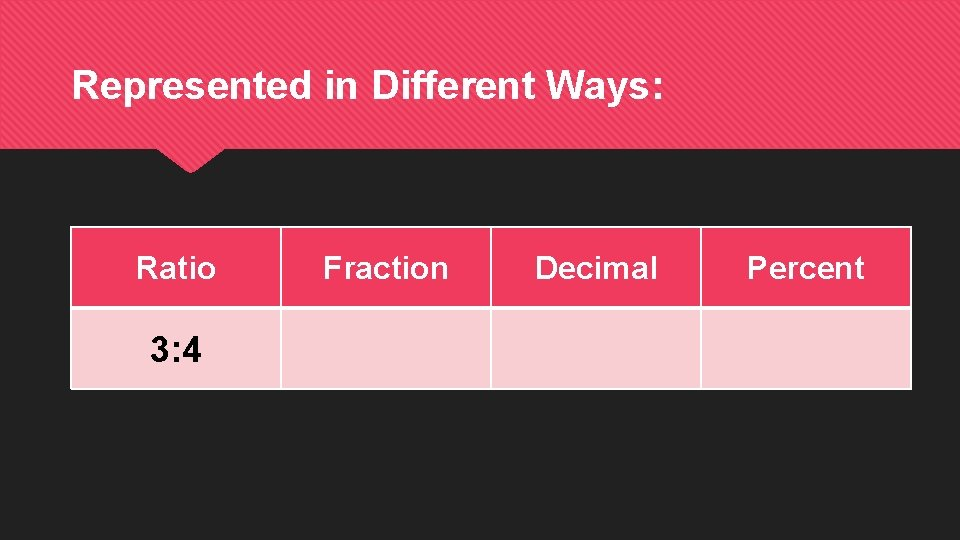Represented in Different Ways: Ratio 3: 4 Fraction Decimal PercentRepresented in Different Ways: Ratio Fraction 3: 4 3/4 Decimal PercentRepresented in Different Ways: Ratio Fraction Decimal 3: 4 3/4 0. 75 PercentRepresented in Different Ways: Ratio Fraction Decimal Percent 3: 4 3/4 0. 75 75%Represented in Different Ways: Ratio 7: 19. 5 Fraction Decimal Percent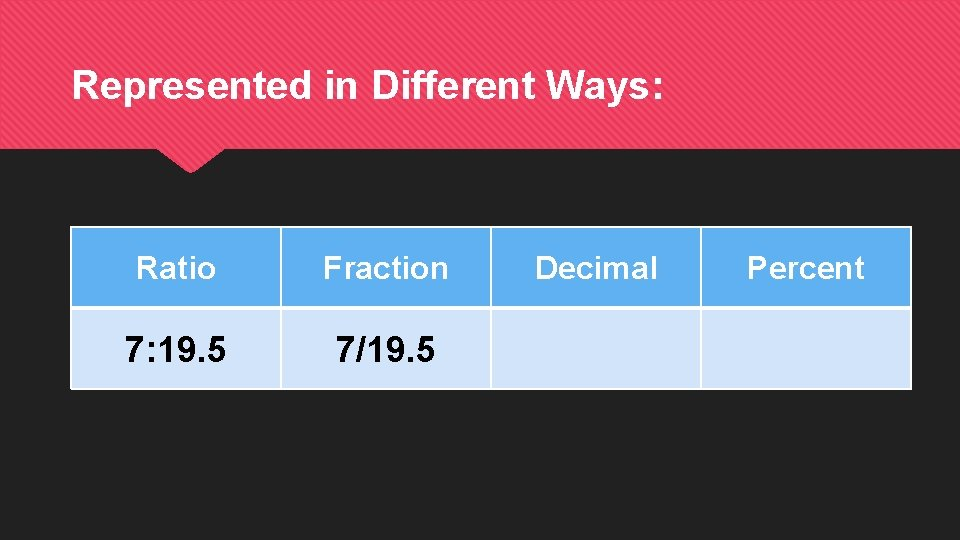Represented in Different Ways: Ratio Fraction 7: 19. 5 7/19. 5 Decimal PercentRepresented in Different Ways: Ratio Fraction Decimal 7: 19. 5 7/19. 5 0. 36 PercentRepresented in Different Ways: Ratio Fraction Decimal Percent 7: 19. 5 7/19. 5 0. 36 36%Equivalent Ratios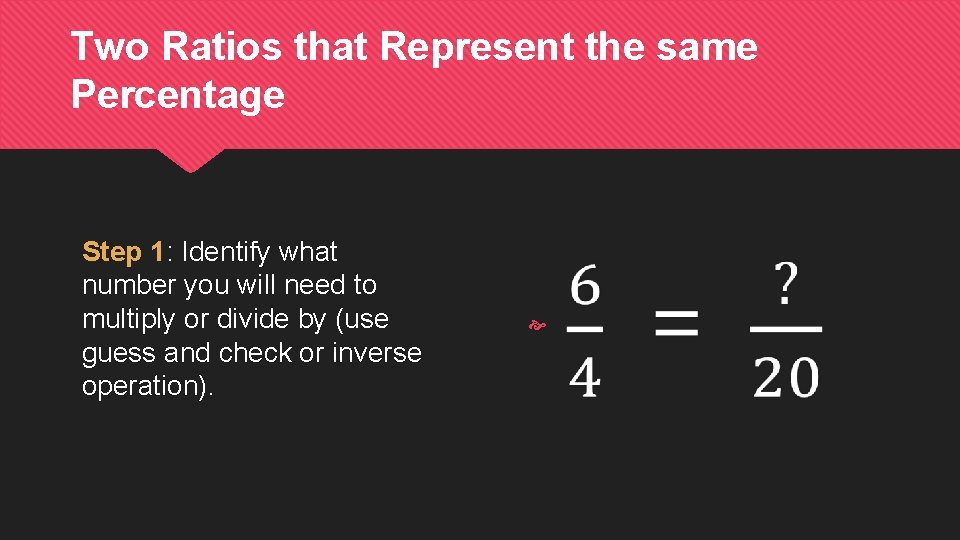Two Ratios that Represent the same Percentage Step 1: Identify what number you will need to multiply or divide by (use guess and check or inverse operation).Two Ratios that Represent the same Percentage Step 1: Identify what number you will need to multiply or divide by (use guess and check or inverse operation).Two Ratios that Represent the same Percentage Step 1: Identify what number you will need to multiply or divide by (use guess and check or inverse operation). Step 2: Multiply or divide to uncover the missing number.Two Ratios that Represent the same Percentage Step 1: Identify what number you will need to multiply or divide by (use guess and check or inverse operation). Step 2: Multiply or divide to uncover the missing number.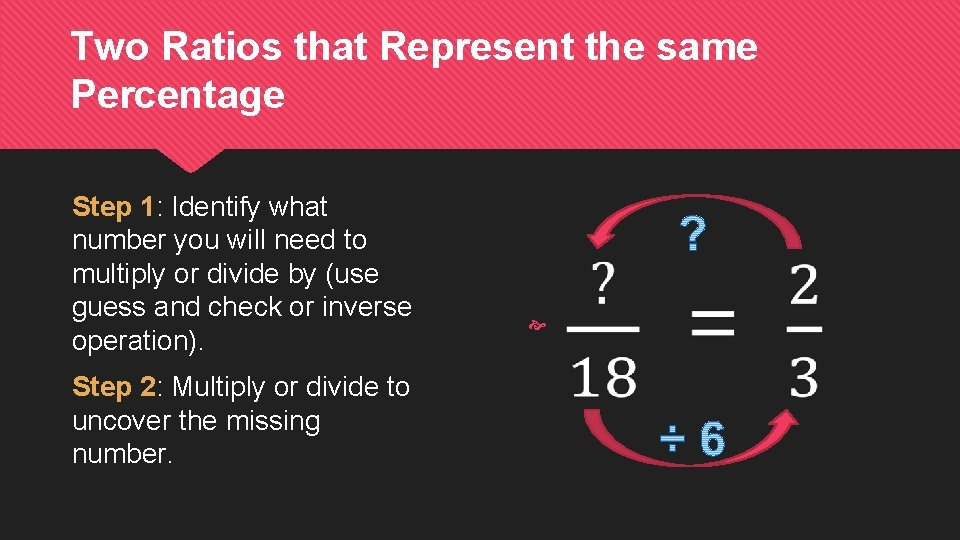Two Ratios that Represent the same Percentage Step 1: Identify what number you will need to multiply or divide by (use guess and check or inverse operation). Step 2: Multiply or divide to uncover the missing number.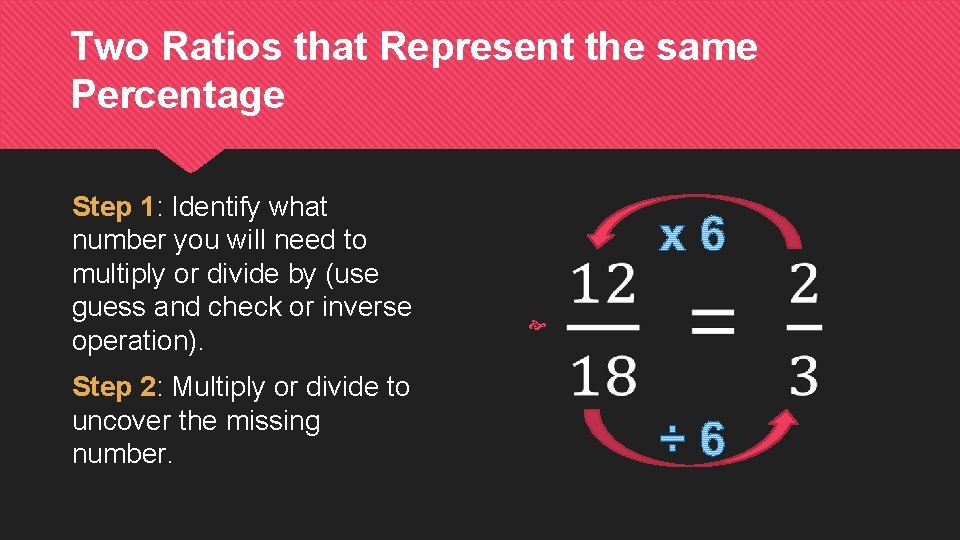Two Ratios that Represent the same Percentage Step 1: Identify what number you will need to multiply or divide by (use guess and check or inverse operation). Step 2: Multiply or divide to uncover the missing number.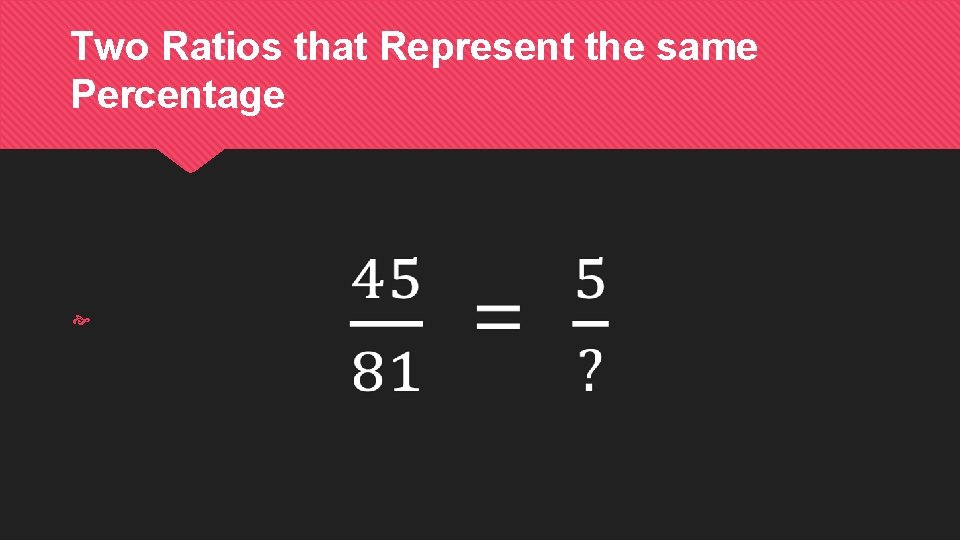Two Ratios that Represent the same PercentageTwo Ratios that Represent the same PercentageTwo Ratios that Represent the same PercentageTwo Ratios that Represent the same PercentageTwo Ratios that Represent the same Percentage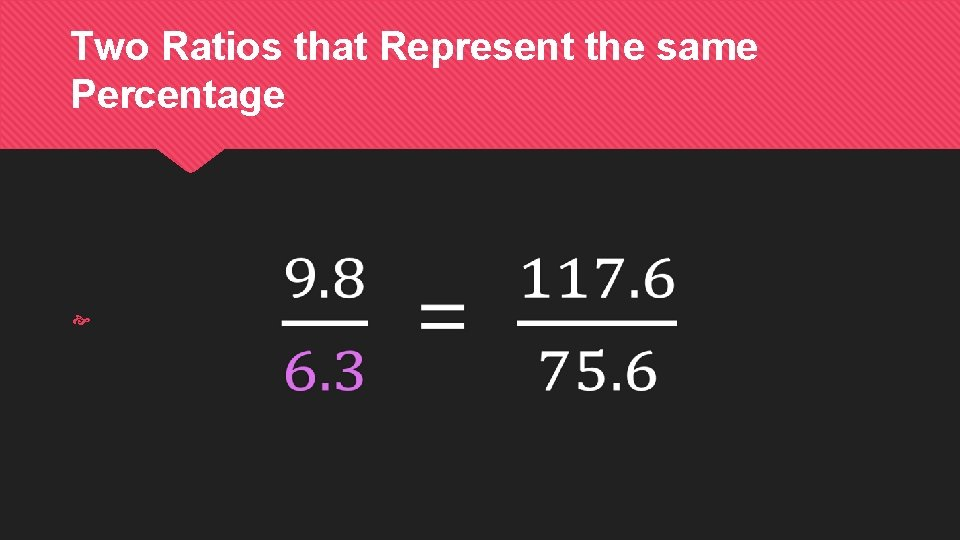Two Ratios that Represent the same Percentage